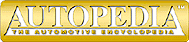The Physics of Racing
Part 6: Speed and Horsepower

These articles were written by Brian Beckman (brianbec@microsoft.com) physicist, and member of No Bucks Racing Club. ©Copyright 1991, Brian Beckman

The title of this month's article consists of two words dear to every racer's heart. This month, we do some ``back of the envelope'' calculations to investigate the basic physics of speed and horsepower (the ``back of the envelope'' style of calculating was covered in part 3 of this series).

How much horsepower does it take to go a certain speed? At first blush, a physicist might be tempted to say ``none,'' because he or she remembers Newton's first law, by which an object moving at a constant speed in a straight line continues so moving forever, even to the end of the Universe, unless acted on by an external force. Everyone knows, however, that it is necessary to keep your foot on the gas to keep a car moving at a constant speed. Keeping your foot on the gas means that you are making the engine apply a backward force to the ground, which applies a reaction force forward on the car, to keep the car moving. In fact, we know a few numbers from our car's shop manual. A late model Corvette, for example, has a top speed of about 150 miles per hour and about 240 hp. This means that if you keep your foot all the way down, using up all 240 hp, you can eventually go 150 mph. It takes a while to get there. In this car, you can get to 60 mph in about 6 seconds (if you don't spin the drive wheels), to 100 mph in about 15 seconds, and 150 in about a minute.

All this seems to contradict Newton's first law. What is going on? An automobile moving at constant speed in a straight line on level ground is, in fact, acted on by a number of external forces that tend to slow it down. Without these forces, the car would coast forever as guaranteed by Newton's first law. You must counteract these forces with the engine, which indirectly creates a reaction force that keeps the car going. When the car is going at a constant speed, the net force on the car, that is, the speeding-up forces minus the slowing-down forces, is zero.

The most important external, slowing-down force is air resistance or drag. The second most important force is friction between the tires and the ground, the so-called rolling resistance. Both these forces are called resistance because they always act to oppose the forward motion of the car in whatever direction it is going. Another physical effect that slows a car down is internal friction in the drive train and wheel bearings. Acting internally, these forces cannot slow the car. However, they push backwards on the tires, which push forward on the ground, which pushes back by Newton's third law, slowing the car down. The internal friction forces are opposed by external reaction forces, which act as slight braking forces, slowing the car. So, Newton and the Universe are safe; everything is working as it should.

How big are the resistance forces, and what role does horsepower play? The physics of air resistance is very complex and an area of vigorous research today. Most of this research is done by the aerospace industry, which is technologically very closely related to the automobile industry, especially when it comes to racing. We'll slog through some arithmetic here to come up with a table that shows how much horsepower it takes to sustain speed. Those who don't have the stomach to go through the math can skim the next few paragraphs.

We cannot derive equations for air resistance here. We'll just look them up. My source is Fluid Mechanics, by L. D. Landau and E. M. Lifshitz, two eminent Russian physicists. They give the following approximate formula: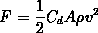The factors in this equation are the following: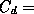coefficient of friction, a factor depending on the shape of a car and determined by experiment; for a late model Corvette it is about 0.30;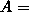frontal area of the car; for a Corvette, it is about 20 square feet;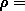Greek letter rho, density of air, which we calculate below;speed of the car.

Let us calculate the density of air using ``back of the envelope'' methods. We know that air is about 79%Nitrogen and 21%Oxygen. We can look up the fact that Nitrogen has a molecular weight of about 28 and Oxygen has a molecular weight of about 32. What is molecular weight? It is the mass (not the weight, despite the name) of 22.4 liters of gas. It is a number of historical convention, just like feet and inches, and doesn't have any real science behind it. So, we figure that air has an average molecular weight ofI admit to using a calculator to do this calculation, against the spirit of the ``back of the envelope'' style. So sue me.

We need to convert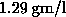to pounds of mass per cubic foot so that we can do the force calculations in familiar, if not convenient, units. It is worthwhile to note, as an aside, that a great deal of the difficulty of doing calculations in the physics of racing has to do with the traditional units of feet, miles, and pounds we use. The metric system makes all such calculations vastly simpler. Napoleon Bonaparte wanted to convert the world the metric system (mostly so his own soldiers could do artillery calculations quickly in their heads) but it is still not in common use in America nearly 200 years later!

Again, we look up the conversion factors. My source is Engineering Formulas by Kurt Gieck, but they can be looked up in almost any encyclopedia or dictionary. There are 1000 liters in a cubic meter, which in turn contains 35.51 cubic feet. Also, a pound-mass contains 453.6 grams. These figures give us, for the density of air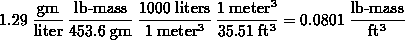This says that a cubic foot of air weighs 8 hundredths of a pound, and so it does! Air is much more massive than it seems, until you are moving quickly through it, that is.

Let's finish off our equation for air resistance. We want to fill in all the numbers except for speed,, using the Corvette as an example car so that we can calculate the force of air resistance for a variety of speeds. We get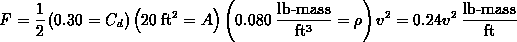We want, at the end, to havein miles per hour, but we needin feet per seconds for the calculations to come out right. We recall that there are 22 feet per second for every 15 miles per hour, giving usNow (this gets confusing, and it wouldn't be if we were using the metric system), a pound mass is a phony unit. A lb-mass is concocted to have a weight of 1 pound under the action of the Earth's gravity. Pounds are a unit of force or weight, not of mass. We want our force of air resistance in pounds of force, so we have to divide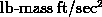by 32.1, numerically equal to the acceleration of Earth's gravity in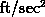, to get pounds of force. You just have to know these things. This was a lot of work, but it's over now. We finally get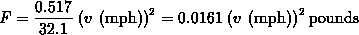Let's calculate a few numbers. The following table gives the force of air resistance for a number of interesting speeds: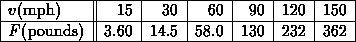We can see that the force of air resistance goes up rapidly with speed, until we need over 350 pounds of constant force just to overcome drag at 150 miles per hour. We can now show where horsepower comes in.

Horsepower is a measure of power, which is a technical term in physics. It measures the amount of work that a force does as it acts over time. Work is another technical term in physics. It measures the actual effect of a force in moving an object over a distance. If we move an object one foot by applying a force of one pound, we are said to be doing one foot-pound of work. If it takes us one second to move the object, we have exerted one foot-pound per second of power. A horsepower is 550 foot-pounds per second. It is another one of those historical units that Napoleon hated and that has no reasonable origin in science.

We can expend one horsepower by exerting 550 pounds of force to move an object 1 foot in 1 second, or by exerting 1 pound of force to move an object 550 feet in 1 second, or by exerting 1 pound of force to move an object 1 foot in 0.001818 seconds, and so on. All these actions take the same amount of power. Incidentally, a horsepower happens to be equal also to 745 watts. So, if you burn about 8 light bulbs in your house, someone somewhere is expending at least one horsepower (and probably more like four or five) in electrical forces to keep all that going for you, and you pay for the service at the end of the month!.

All this means that to find out how much horsepower it takes to overcome air resistance at any speed, we need to multiply the force of air resistance by speed (in feet per second, converted from miles per hour), and divide by 550, to convert foot-lb/sec to horsepower. The formula is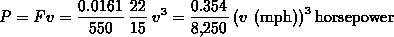and we get the following numbers from the formula for a few interesting speeds.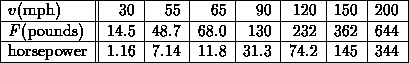I put 55 mph and 65 mph in this table to show why some people think that the 55 mph national speed limit saves gasoline. It only requires about 7 hp to overcome drag at 55 mph, while it requires almost 12 hp to overcome drag at 65. Fuel consumption is approximately proportional to horsepower expended.

More interesting to the racer is the fact that it takes 145 hp to overcome drag at 150 mph. We know that our Corvette example car has about 240 hp, so about 95 hp must be going into overcoming rolling resistance and the slight braking forces arising from internal friction in the drive train and wheel bearings. Race cars capable of going 200 mph usually have at least 650 hp, about 350 of which goes into overcoming air resistance. It is probably possible to go 200 mph with a car in the 450-500 hp range, but such a car would have very good aerodynamics; expensive, low-friction internal parts; and low rolling resistance tires, which are designed to have the smallest possible contact patch like high performance bicycle tires, and are therefore not good for handling.